# Arranging Numbers in Ascending Order (1)

In this worksheet, students place four numbers in increasing order.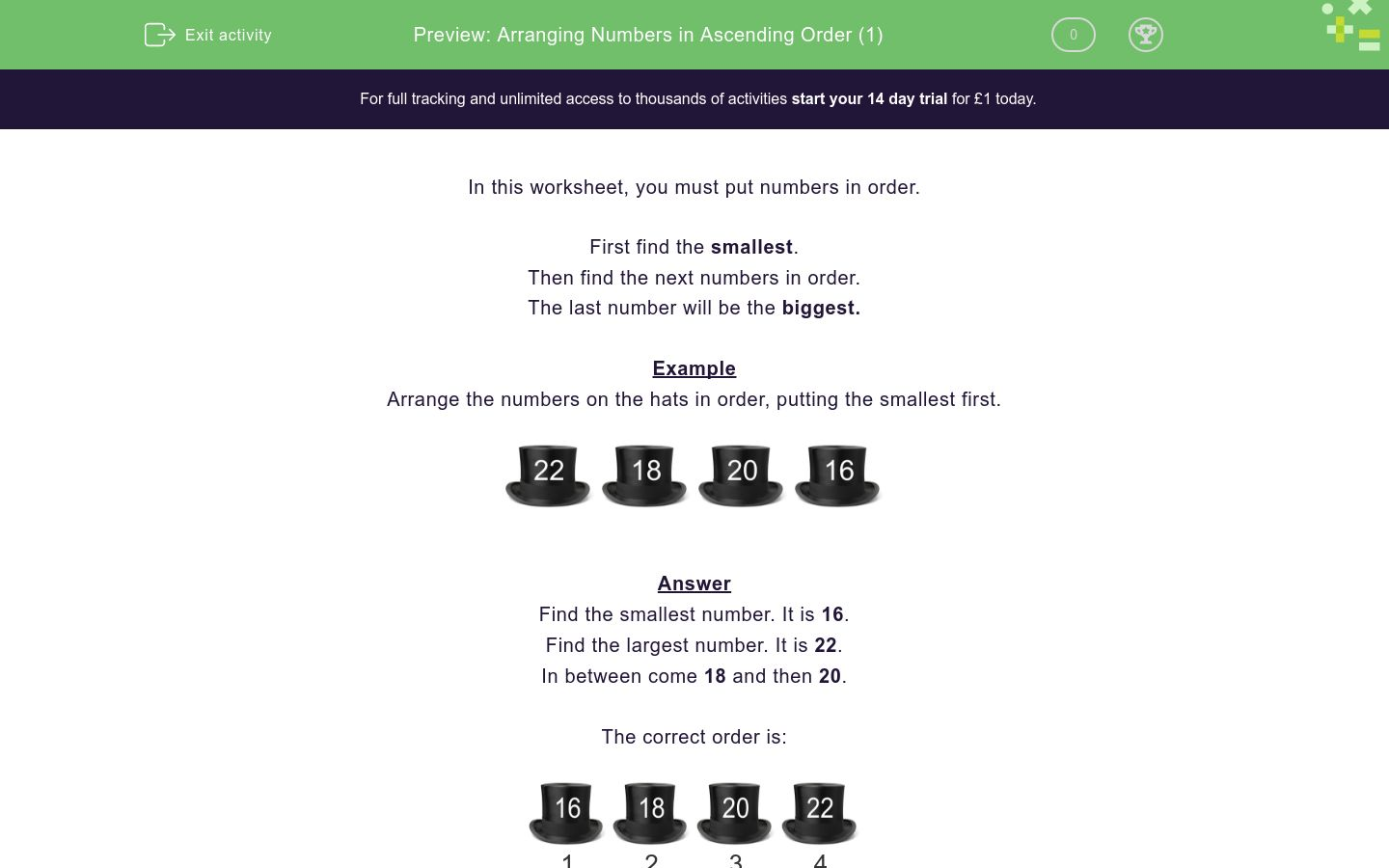Key stage:  KS 1

Curriculum topic:   Number: Number and Place Value

Curriculum subtopic:   Order/Compare Numbers to 100

Difficulty level:### QUESTION 1 of 10

In this worksheet, you must put numbers in order.

First find the smallest.

Then find the next numbers in order.

The last number will be the biggest.

Example

Arrange the numbers on the hats in order, putting the smallest first.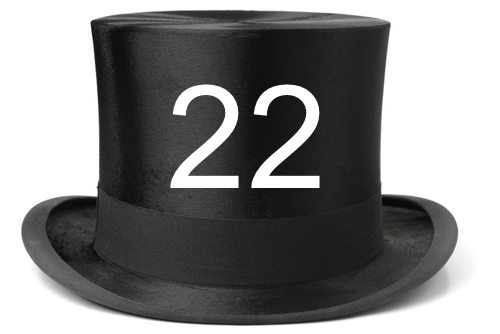Find the smallest number. It is 16.

Find the largest number. It is 22.

In between come 18 and then 20.

The correct order is:1 2 3 4

Arrange the numbers on the hats in order, putting the smallest first.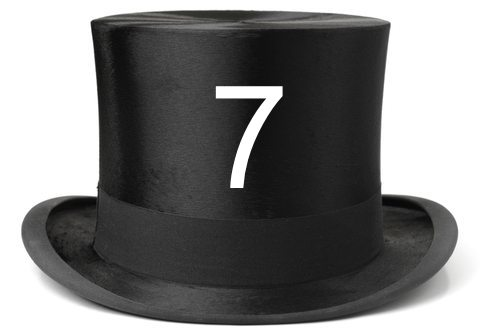## Column B

1
11
2
7
3
5
4
9

Arrange the numbers on the hats in order, putting the smallest first.## Column B

1
20
2
16
3
18
4
22

Arrange the numbers on the hats in order, putting the smallest first.## Column B

1
5
2
4
3
6
4
3

Arrange the numbers on the hats in order, putting the smallest first.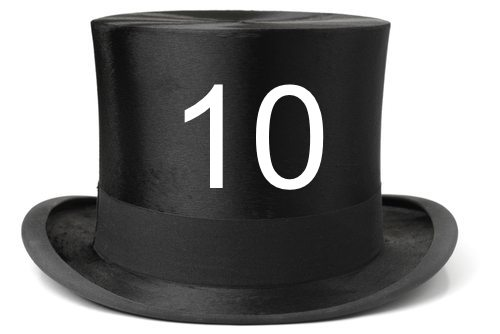## Column B

1
10
2
8
3
11
4
9

Arrange the numbers on the hats in order, putting the smallest first.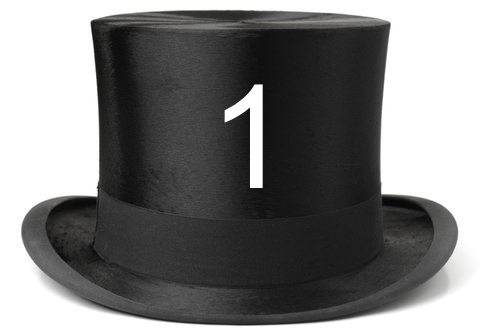## Column B

1
5
2
3
3
1
4
7

Arrange the numbers on the hats in order, putting the smallest first.## Column B

1
22
2
23
3
25
4
24

Arrange the numbers on the hats in order, putting the smallest first.## Column B

1
9
2
1
3
13
4
5

Arrange the numbers on the hats in order, putting the smallest first.## Column B

1
14
2
1
3
20
4
17

Arrange the numbers on the hats in order, putting the smallest first.## Column B

1
2
2
4
3
3
4
1

Arrange the numbers on the hats in order, putting the smallest first.## Column B

1
18
2
22
3
16
4
20
• Question 1

Arrange the numbers on the hats in order, putting the smallest first.## Column B

1
5
2
7
3
9
4
11
EDDIE SAYS
The smallest is 5.
Then come 7 and 9 in order.
The biggest is 11.
• Question 2

Arrange the numbers on the hats in order, putting the smallest first.## Column B

1
16
2
18
3
20
4
22
EDDIE SAYS
The smallest is 16.
Then come 18 and 20 in order.
The biggest is 22.
• Question 3

Arrange the numbers on the hats in order, putting the smallest first.## Column B

1
3
2
4
3
5
4
6
EDDIE SAYS
The smallest is 3.
Then come 4 and 5 in order.
The biggest is 6.
• Question 4

Arrange the numbers on the hats in order, putting the smallest first.## Column B

1
8
2
9
3
10
4
11
EDDIE SAYS
The smallest is 8.
Then come 9 and 10 in order.
The biggest is 11.
• Question 5

Arrange the numbers on the hats in order, putting the smallest first.## Column B

1
1
2
3
3
5
4
7
EDDIE SAYS
The smallest is 1.
Then come 3 and 5 in order.
The biggest is 7.
• Question 6

Arrange the numbers on the hats in order, putting the smallest first.## Column B

1
22
2
23
3
24
4
25
EDDIE SAYS
The smallest is 22.
Then come 23 and 24 in order.
The biggest is 25.
• Question 7

Arrange the numbers on the hats in order, putting the smallest first.## Column B

1
1
2
5
3
9
4
13
EDDIE SAYS
The smallest is 1.
Then come 5 and 9 in order.
The biggest is 13.
• Question 8

Arrange the numbers on the hats in order, putting the smallest first.## Column B

1
1
2
14
3
17
4
20
EDDIE SAYS
The smallest is 1.
Then come 14 and 17 in order.
The biggest is 20.
• Question 9

Arrange the numbers on the hats in order, putting the smallest first.## Column B

1
1
2
2
3
3
4
4
EDDIE SAYS
The smallest is 1.
Then come 2 and 3 in order.
The biggest is 4.
• Question 10

Arrange the numbers on the hats in order, putting the smallest first.## Column B

1
16
2
18
3
20
4
22
EDDIE SAYS
The smallest is 16.
Then come 18 and 20 in order.
The biggest is 22.
---- OR ----

Sign up for a £1 trial so you can track and measure your child's progress on this activity.

### What is EdPlace?

We're your National Curriculum aligned online education content provider helping each child succeed in English, maths and science from year 1 to GCSE. With an EdPlace account you’ll be able to track and measure progress, helping each child achieve their best. We build confidence and attainment by personalising each child’s learning at a level that suits them.

Get started